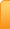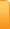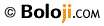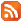Hand Multiplication by N. S. MurtyChannelsAnalysis
Cartoons
Education
Environment
Opinion
Photo Essays

Random Thoughts

Architecture
Astrology
Ayurveda
Buddhism
Cinema
Culture
Festivals
Hinduism
History
People
Places
Sikhism
Spirituality

Health
Parenting
Perspective
Recipes
Society
Teens
Women

## Creative Writings

Book Reviews
Computing
Humor
Individuality
Literary Shelf
Memoirs
Quotes
Stories
Travelogues
Workshop

 Education Share This Page Hand Multiplication by N. S. MurtyContinued from “Mathematics Made Easy” I Well in the beginning I promised you multiplication tables using the fingers of your hands and without the use of calculator up to 20x20. I did not forget. In fact if you master the technique you never need a calculator for any multiplication and you never need to commit tables to memory. But first remember few important things. Since we are using only the fingers of our hands which are finite and as the numbers grow we have to assign some values to the fingers depending upon the growth. That is all the care you have to take and remember. Let us represent the fingers of the left hand as LL, LR, LM, LI and LT which respectively mean Little, Ring, Middle, Index and Thumb of the Left Hand. Similarly, RL, RR, RM, RI and RT represent respectively Little, Ring, Middle, Index and Thumb of the Right Hand. Cycles In the first cycle we assign values 6 to 10 to LL, LR, LM, LI, LT and RL, RR, RM, RI, and RT. Our tables start from 6x6 and we assume you know the values up to this point. The procedure and the values Suppose you need to find the values from 6x6 to 10x10 in this cycle you can give it in a trice if you practice well. Step1 Join the fingers representing the multiplicands. That means 1) if you need 6x8 join LL and RM, 2) if you need 7x9 join LR and RI, 3) if you need 8x9 join LM and RI and so on. Value 1 All the fingers joined and lying below represent 10s. In the first example given of 6x8, LL, RL, RR, and RM represents 10s In the second case, LL, LR, RL, RR, RM and RI represent 10s In the third case, LL, LR, LM, RL, RR, RM. and RI represent 10s Step 2 Multiply the number of free fingers on the left with the number of free fingers on the right. There may be free fingers on one or both hands. They represent units if they are there, otherwise zero. In case 1, LR to LT are free (4 fingers on the left hand), and RI and RT (2 fingers on the right hand) are free. In case 2, 3 on the left and 1 on the right is free. In case 3, 2 on the left and 1 on the right are free. Value 2: Find the product of number of free fingers on both hands. If the product exceeds 10 carry forward 1 to the Tens place. Step 3 Add the tens and the value of step 2 to get the final value. In case 1, we have 4 tens and 4x2=8 units, therefore 6x8=48 In case 2, we have 6 tens and 3x1=3 units, therefore, 7x9=63 In case 3, we have 7 tens and 2x1=2 units, therefore, 8x9=72. Please go through from 6x6 to 10x10, we get every value with ease. It seems sometimes, if god has given us 10 fingers to two hands to use decimal system. Isn’t it? II Now comes the vantage over calculator. Let us suppose we want products between 11x11s to 15x15s. Remember we skipped in between products 6x11s to 6x15s. I will come to it after this to make a point clear to you. So bear with me for few minutes. Now, let us turn to our problem of 11x 11s to 15x15s. As we have only ten fingers, and as we go up we associate larger values to each finger, correspondingly we have to change the values of fingers joined and below, as also the values of the free fingers. How we change is all that you need to pay your attention to. If you got it, the answer is very very simple. Just look. When we are multiplying figures from 6x6 to 10x 10, we started with values above 5 for each hand. Then the sum of these two is 10. So we assigned to the fingers joined and below them a value of 10. Now for values between 11x11 and 15 x15, we start giving to each finger on the right and left a value above 10. So what value should we give by analogy to the fingers joined and below them now? Right. 10+10= 20. And what about the values of free fingers? You must add 5 to the number of free fingers on the right as well as left. Let us do it by example. Example 1. Suppose you want 12x12 What fingers do you join? LR and RR. So there are 4 fingers joined and below, their value is 4x20 = 80. Then there are 3 free fingers each on two hands. So their value is 5+3 in each case and their product is 64. Now adding 64 to 80 we get 144 which is exactly what it is. Example 2. Take for example 14 x 12 We join LI and RR. There are 6 fingers joined or below. Their value is 120. And 1 free finger on the left, its value is 6 and 3 free fingers on the right, their value is 8 and the product is 6x8=48. Adding 120 + 48= 168 and 14x12 is that. And now you can master up to 15x15. Let us get back to the problem I left out deliberately. That is from 6x11s to 6x15s. In the cases I described above, the range of multiplication in both the hands is same. That means, we are assigning the same set of values, say from 6 to 10 or 11 to 15 for the fingers of both hands. There by they are evenly matched. But when you multiply 6 by 11, you have to take care while assigning values to the joined fingers and below them, and the free fingers. (Following the order that the order of multiplication that the left number is represented by the left hand and the right number by the right hand I am giving the following explanation.) Whenever a large number is multiplied by a small number, we can say that the large number is pulled down or the small number is pulled up. Applying the same thing, we assign the values as follows: All fingers joined and below them will be assigned values 10+5 = 15. (Please note one hand starts with 5+ and another with 10+ so the fingers joined and below are assigned 15) 1. Free fingers opposite to the lesser number will be units and to the free fingers opposite to the larger number we add 5 2. There is no change in the other procedure. Let us do by example to be very clear. Example 3: Take for example 7x 13. As usual we join LR and RM. We have 5 fingers joined or below. Each has a value of 15 and so their total is 15x5= 75. Now since left hand is lesser number, and there are 3 free fingers, their value is 3+5= 8 whereas the right hand has 2 free fingers and their value is units only. Thereby their product is 8x2=16. Then add 75 +16 to get 91 which is 7x13. Example 4: 6x14 We join LL and RI. There are 5 fingers joined and below. Their value is 5x15= 75. For the left hand there are 4 free fingers but their value is 4+5=9 where as there is only 1 free finger on the right and its value is 1. Their product is 9. The final value is 75 + 9=84. And now you have mastered from 6x6 to 15 x15. Master this before you venture into the higher plane. Good luck. Share This: 23-Sep-2018 More by :  N. S. Murty Top | Education Views: 1381      Comments: 0 Name * Email ID  (will not be published) Comment * Characters Verification Code* Can't read? Reload Please fill the above code for verification.RSS Feed Home | Privacy Policy | Disclaimer | Site Map